# Set in Java

Challenge Inside! : Find out where you stand! Try quiz, solve problems & win rewards!
Learn via video courseJava Course - Mastering the Fundamentals
By Tarun LuthraFree
5Enrolled: 1000Java Course - Mastering the Fundamentals
Tarun LuthraFree
5
Enrolled: 1000

## Overview

A collection of unique elements is termed a set. Java offers a set interface that can be used to store distinct values. It provides all the functionalities so that you can perform operations like union, intersection, etc.

## Introduction to Set in Java

We are back with yet another classical mathematical collection called Set.

Set is a collection of distinct elements. To simplify further, each element in a set is unique. Strictly speaking, a set cannot have duplicate values.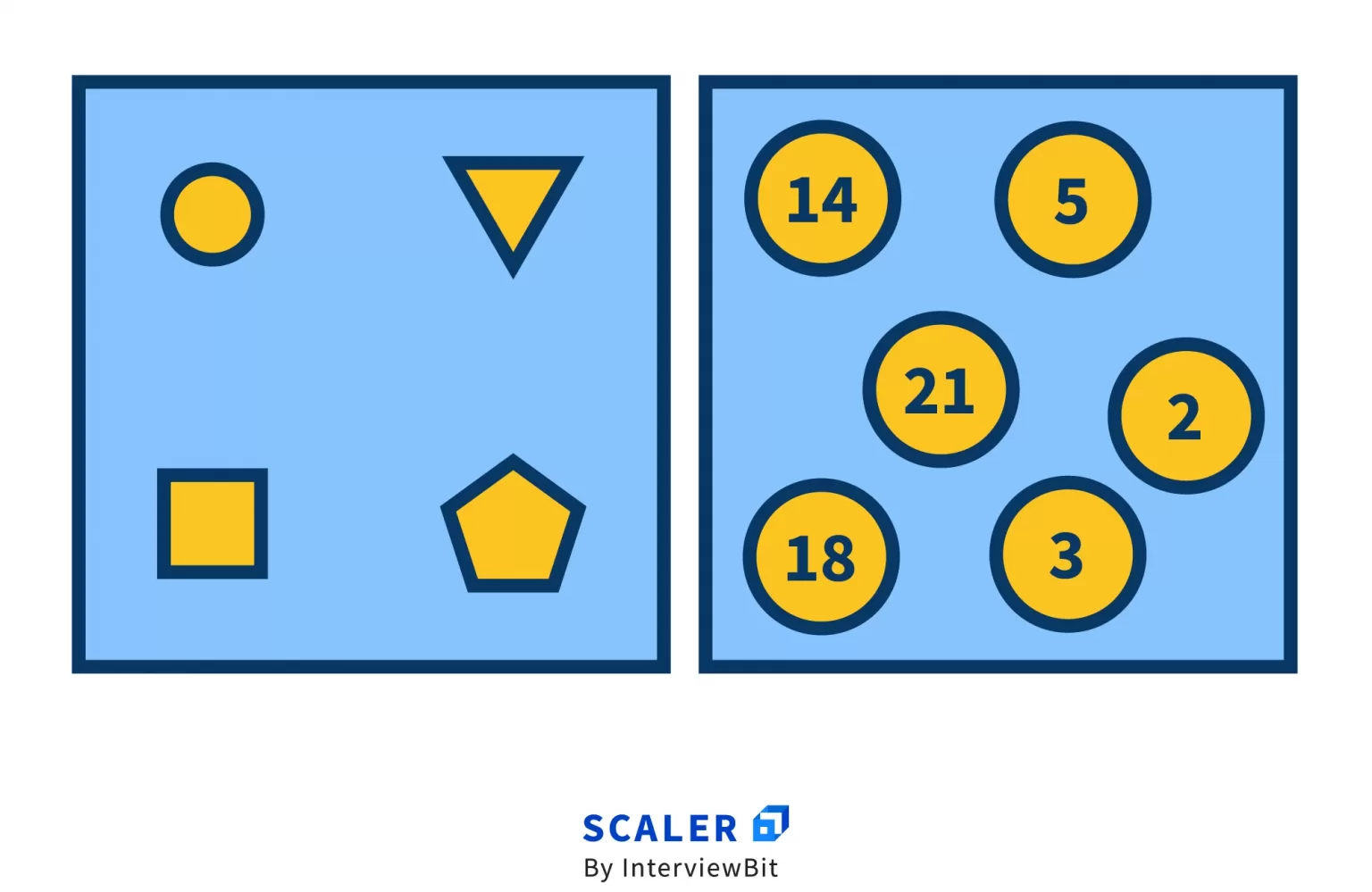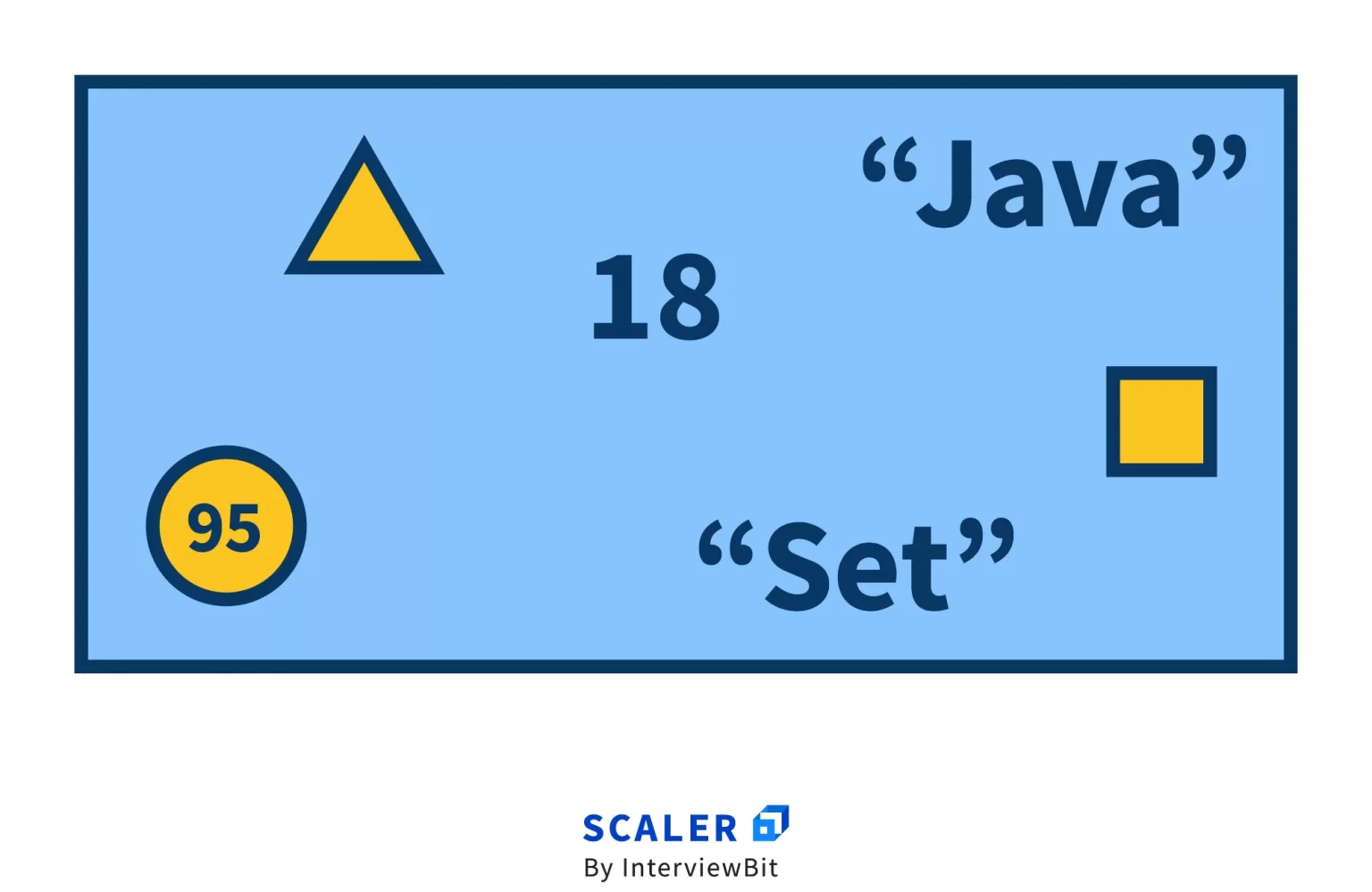For example, all positive numbers i.e., [1, 2, 3, 4, … infinity] belong to the set of natural numbers.

Set has a lot of applications in programming. For example, finding unique numbers in a list, graph theory, etc.

## How to Create a Set in Java?

set in Java is represented as an interface (it is an abstract type that defines the behaviors that a class must implement). It is a part of the Collections package. It extends the Collection interface because, at the heart of it, Set is just a Collection of objects with some special properties.

Internally, Set uses a Map to store the elements. Map is an interface in Java that is used to represent key-value pairs. To simplify, each key in a map is associated with a corresponding value. A map stores unique keys in it, just like a set. A key’s corresponding value can be updated in a map, but it will never have multiple entries for a key.

Whenever an element is added in a set, internally it is added in the map as the key and its value is set as “present”. If an element is added again, it can directly add it to the map and need not worry about duplicates since the map ensures the uniqueness of keys out of the box.

A set in Java can be created in the following way.

<Integer> is a Java generic, which means we want to create a set of integers. Similarly, we can create a set of strings as follows.

Here, HashSet is the class that implements the Set interface. Think of it as a flavour of a Set. Just like there are different kinds of flavours of an ice-cream, we have different flavours of a Set in Java.

Each flavour has a different taste i.e., the way it represents a Set. The other most common flavours that are used in Java are LinkedHashSet and TreeSet.

HashSet is the most commonly used flavour of Set in Java. Internally, it uses HashMap which is a flavour of Map. HashMap uses hashing and hashtable to store the elements. It is the fastest in terms of addition, traversal as well as retrieval. The order of insertion is not maintained in HashSet.

LinkedHashSet is similar to HashSet, other than the fact that it maintains the order of insertion. That means the order of iteration of elements is the same as the order in which they were inserted into the set.

TreeSet has a very unique use case. It maintains the elements of the set in their natural order. For example, if you iterate a TreeSet of integers, the iteration will start from the smallest integer up to the largest integer of the set. Internally, it uses the TreeMap flavour of Map. It is called TreeMap because it uses a Tree (Balanced Search Tree) internally to store the elements.

## Operations in Java Sets

Mathematically, 3 fundamental operations can be applied on a set to create new sets.

For illustration, let us take 2 sets, A: [2, 1, 9, 6, 3] and B: [1, 8, 7, 9, 5].

1. Union: This operation returns elements that are present in either of the two sets. It is represented by ∪.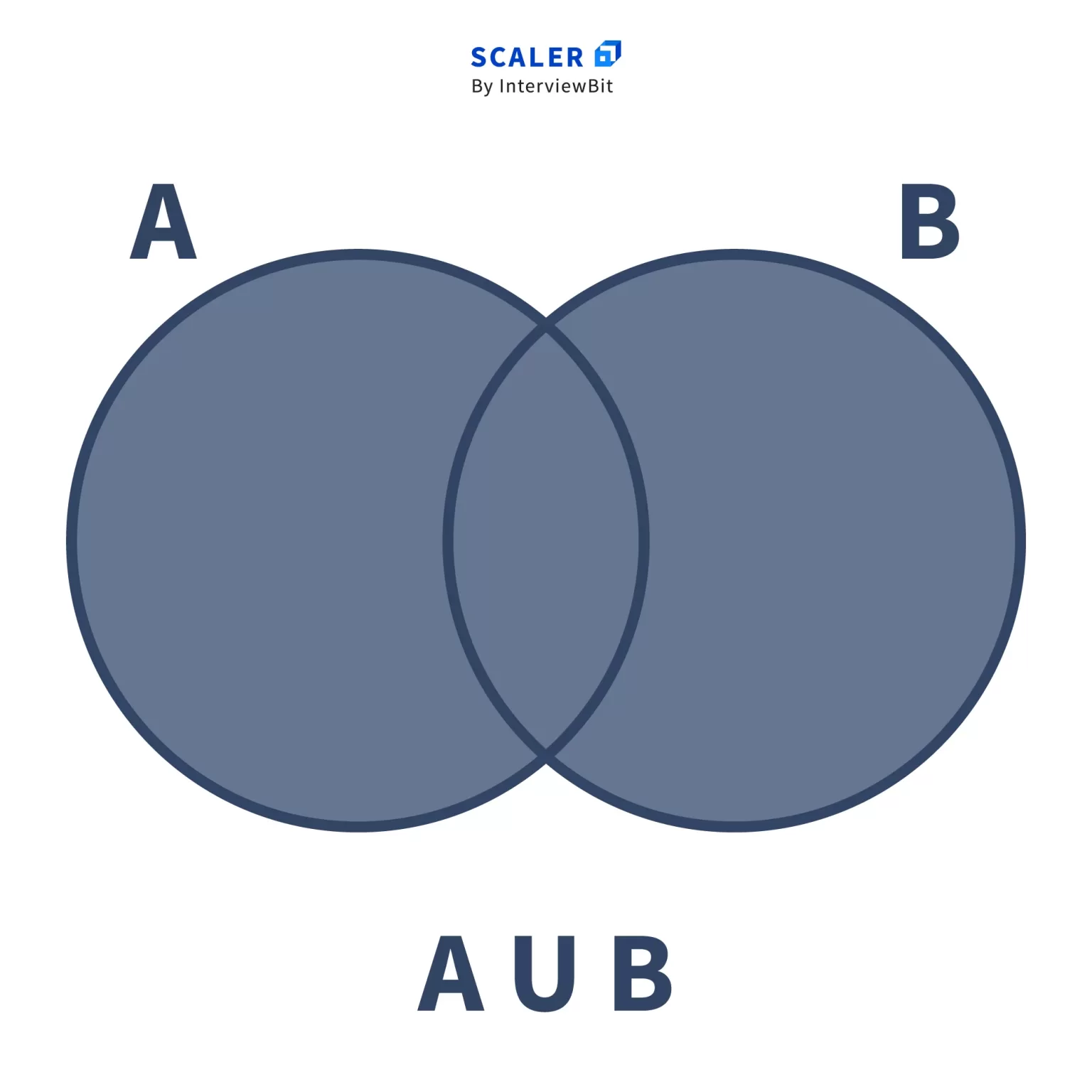It’s similar to an OR operation: return all elements which are present in either set A or B.

This is not a relative operation i.e.

A ∪ B = B ∪ A

So, A ∪ B = [1, 2, 3, 5, 6, 7, 8, 9]

2. Intersection: This operation returns elements that are present in both sets. It is represented by ∩.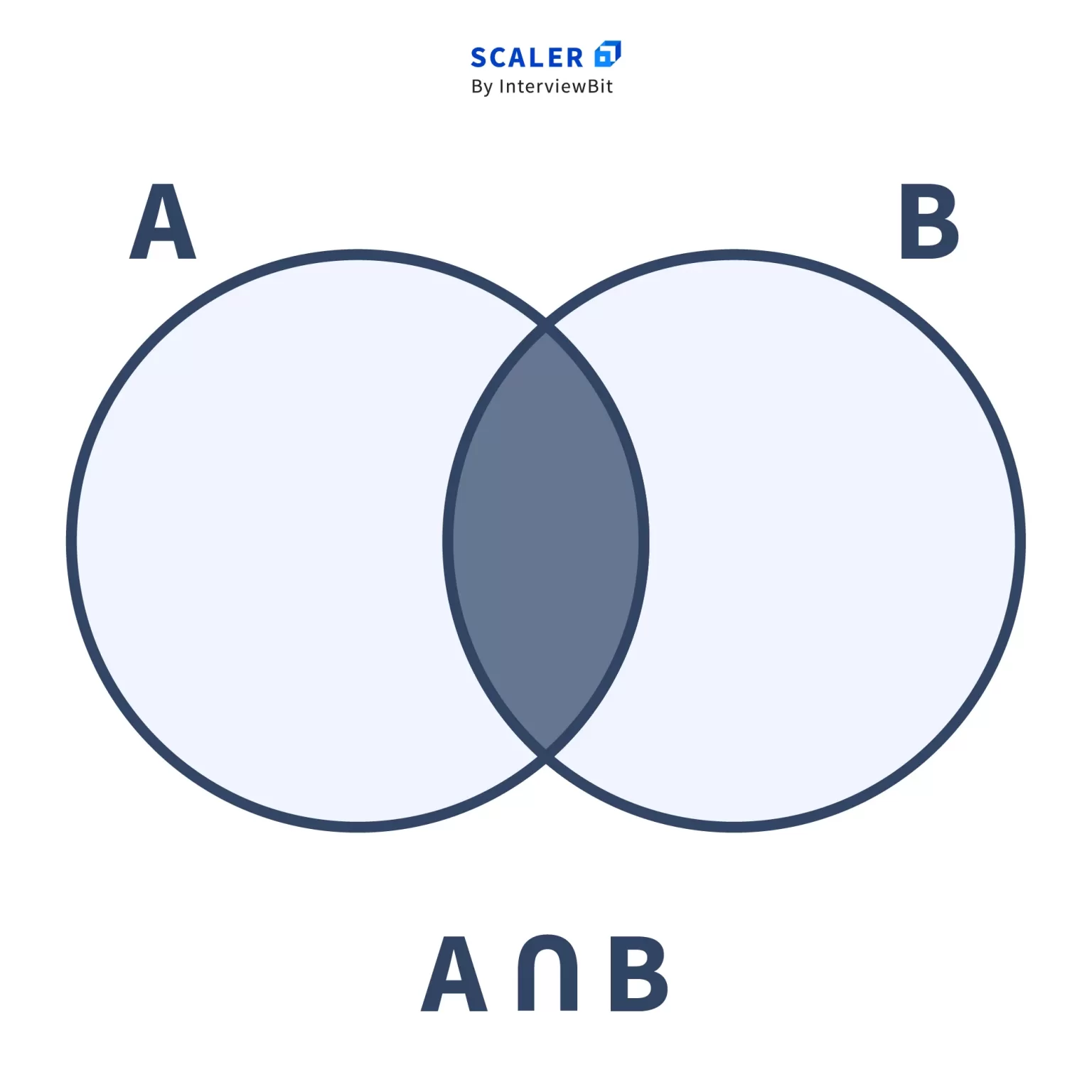It’s similar to an AND operation: return all elements which are present in both sets A and B.

This is also not a relative operation i.e.

A ∩ B = B ∩ A

So, A ∩ B = [1, 9]

3. Complement: It returns elements of the current set which are not present in the other set. It is represented by ∁.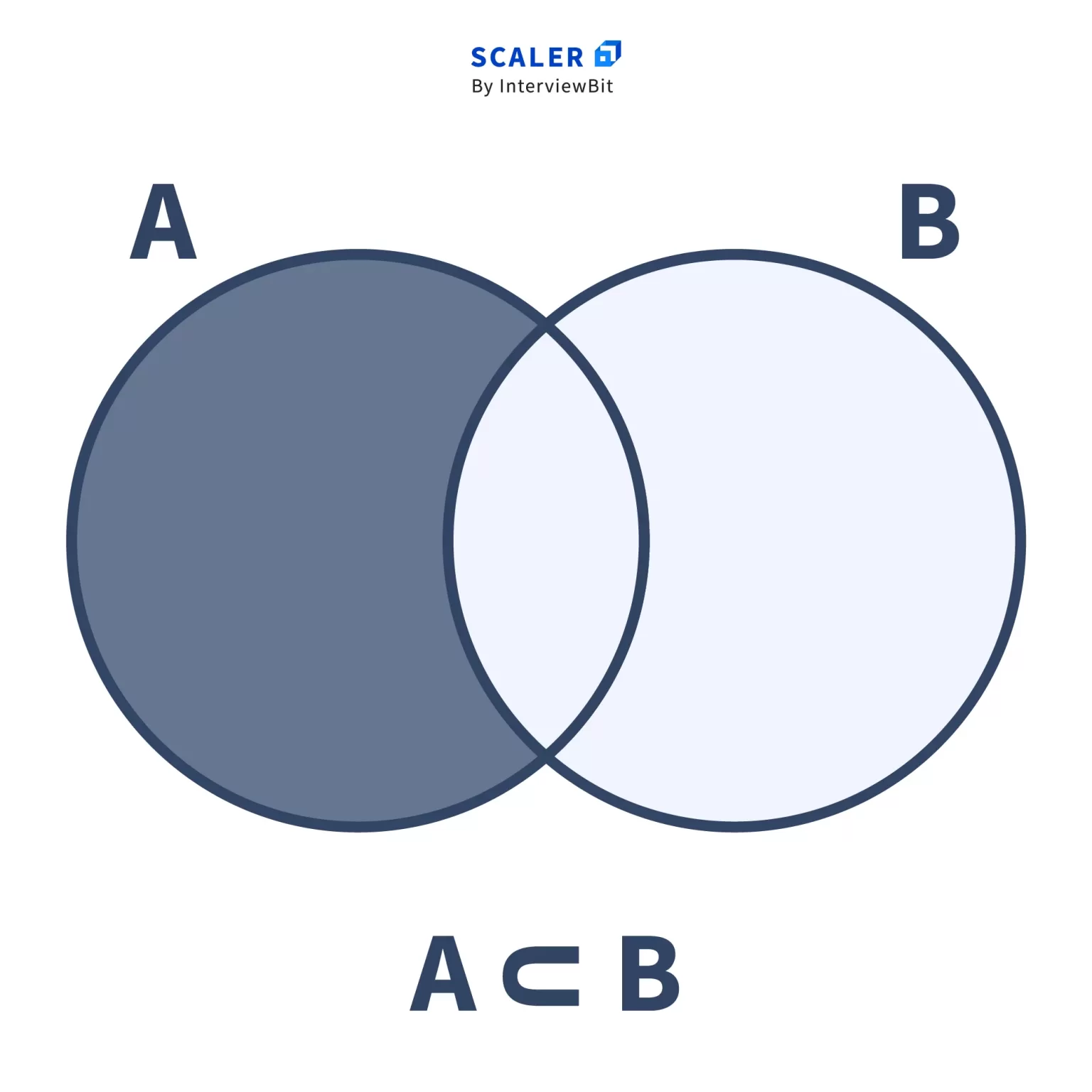It’s similar to a SUBTRACT operation: returns all elements of A which are not present in B.

Unlike Union and Intersection, Complement is a relative operation, i.e.

A ∁ B may or may not be equal to B ∁ A.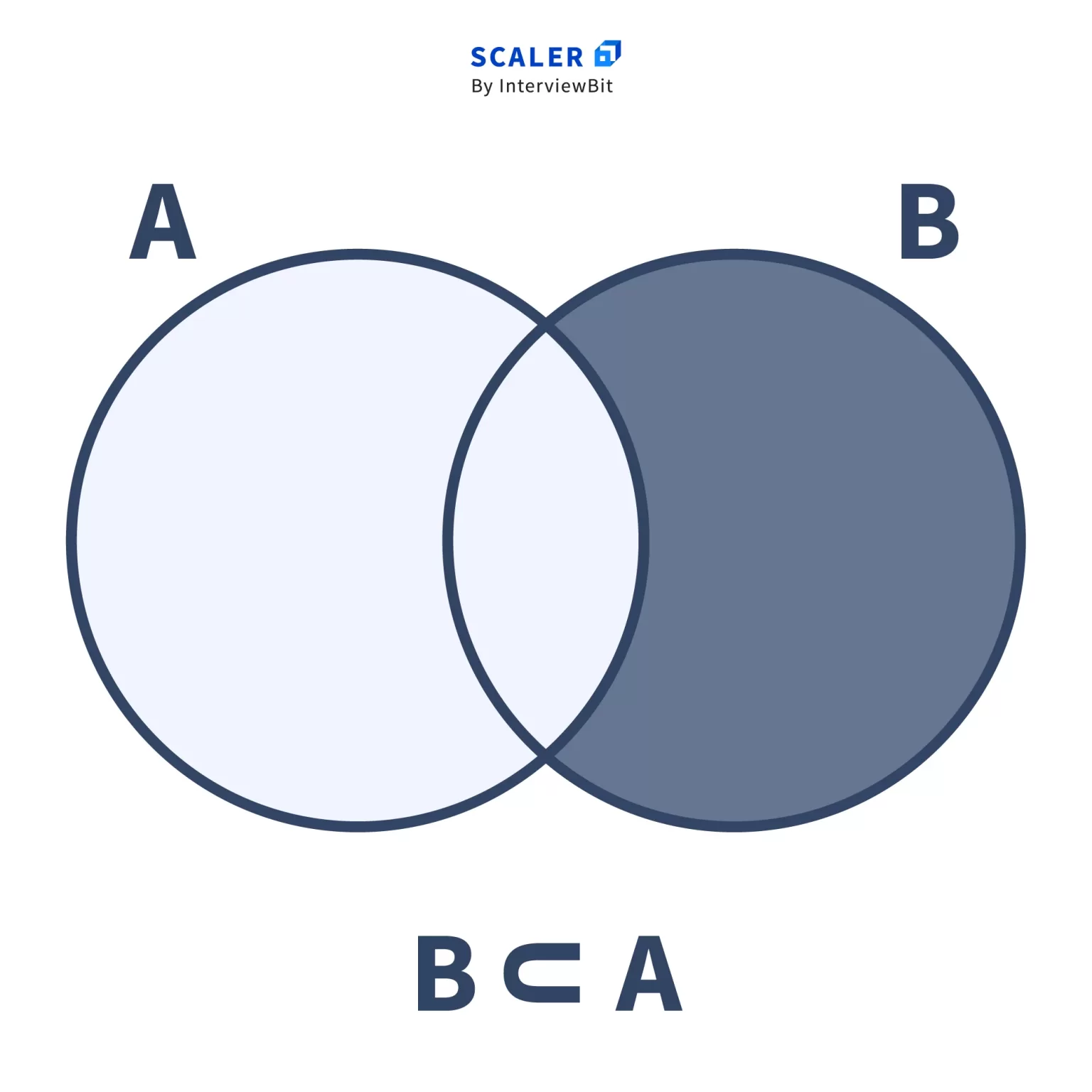So, A ∁ B = [2, 3, 6]

and, B ∁ A = [5, 7, 8]

Food for thought: Can you think of a situation where A Complement B will be equal to B Complement A? 😛

## Set Methods in Java

The Set interface in Java abstracts the different types of operations that can be performed on a set. Let’s discuss them one by one.

A little heads up, all code snippets use the HashSet flavour of Set, and hence, the time complexities (worst case) mentioned below are for the HashSet flavour only.

The add function adds an element to a set. If the element is already present in the set, the operation becomes void i.e., nothing happens.

### Syntax:

Parameters: e, the element to be added Returns: true if the set did not already contain the element e Time Complexity: O(1)

Here, E is the type of the set. In the below example, Set is a set of type Integer.

Output:

The addAll function adds all the elements of a collection to a set.

### Syntax:

? is a wildcard in Java. ? extends E represents a collection of E or sub-types of E.

For example,

Parameters: c, the collection to be added

Returns: true, if the set was modified by this operation i.e., at least one element was added from c Time Complexity: O(size) where size is the number of elements in the collection c

The parameter c can be of any type of Collection. It can be a Set, a List, or any user-defined class which implements the Collection interface. Remember, Collection is an interface that just defines the behaviours that all collection classes must implement.

Output

Oh, hang on. Did you notice something here? That’s right. The addAll function is analogous to the Union operation we defined earlier. It performs the Union operation on both sets.

### 3. clear()

The clear function empties the set i.e., it removes all elements from the set. It doesn’t delete the set itself, just the elements from it.

### Syntax:

The function does not have any input parameters and does not return anything. Time Complexity: O(size) where size is the number of elements in the set

Output

### 4. contains()

The contains function checks whether an element is present in a set.

### Syntax:

Parameters: o, the element whose presence needs to be checked Returns: true, if the element is present in this set Time Complexity: O(size) where size is the number of elements in the set

The equivalence of two elements is checked using the equals function that is part of every object in Java.

So, contains returns true if and only if there is an element e in the set such that

Objects.equals(e, o)

Output

### 5. containsAll()

The containsAll function checks whether a collection of elements is present in a set.

Just like addAll is used to add a collection to a set, containsAll is used to check whether the set contains all elements of a collection.

This function will return false even if a single element of the collection is not found in the set.

### Syntax:

Parameters: c, the collection to be checked for containment in this set.

Returns: true, if all the elements of the collection are present in this set

Time Complexity: O(cSize * setSize) where cSize and setSize are the number of elements in the collection c and the set respectively

Output

### 6. hashCode()

Let us first define hashCode for any object in Java.

Every class in Java extends the Object class by default. There are two built-in methods in the Object class, equals, and hashCode.

The equals method compares an object with any other object for equality. The hashCode method returns an integer hash value of an object.

According to the creators of Java, if two objects are equal according to the equals method, then their hash codes must be equal as well.

So for a set, the hashCode function returns an integer value which is the sum of the hashCode values of all the elements of the set.

If a set allows null values, the hashCode of null is defined to be zero.

This definition of hashCode for set will ensure that for two sets s1 and s2, if s1.equals(s2), then s1.hashCode() == s2.hashCode() will also be true, which is required by the general contract of hashCode for any class in Java.

### Syntax:

Returns: the hash code value of the set

Time Complexity: O(size) where size is the number of elements in the set

Output

### 7. isEmpty()

The isEmpty function checks whether a set is empty or not i.e., whether it has no elements.

### Syntax:

Returns: true if the set contains no elements.

Time Complexity: O(1)

Output

### 8. iterator()

Let us define what an iterator is.

An iterator is an object which can be used to traverse iterables. Iterables are objects which can be iterated or in simple words, traversed one by one. It has some useful functions for traversing iterables.

next() is used to get the next element in the iteration.

hasNext() is used to check if the iteration has any more elements left.

remove() is used to delete the last element returned by the iterator from the underlying collection.

The iterator function returns the iterator of a set, which can be used to retrieve elements from a set, one at a time.

### Syntax:

Returns: an iterator of the set

Time Complexity: O(1)

Output

### 9. remove()

The remove function removes an element from a set if it is present. If the element is not present in the set, the operation becomes void i.e., nothing happens.

### Syntax:

Parameters: o, the element to be removed

Returns: true, if the set contained the element o

Time Complexity: O(size) where size is the number of elements in the set

The element is removed if and only if there is an element e in the set such that

Objects.equals(e, o)

### 10. removeAll()

The removeAll function removes a collection of elements from a set.

### Syntax:

Parameters: c, the collection of elements to be removed

Returns: true, if the set was modified by this operation i.e., at least one element was removed from this set

Time Complexity: O(cSize * setSize) where cSize and setSize are the number of elements in the collection c and the set respectively

Output

This is exactly like the Complement operation we saw earlier. We are removing all the elements of the collection c from the current set. To simplify, we are just Subtracting all elements of c from the current set.

### 11. retainAll()

The retainAll function retains only the elements of the set that are present in the specified collection. In other words, the elements that are present in both the set and the specified collection are kept, the rest are removed.

### Syntax:

Parameters: c, the collection whose elements have to be retained Returns: true, if the set was modified after this operation i.e., any element was removed from the set. Time Complexity: O(cSize * setSize) where cSize and setSize are the numbers of elements in the collection c and the set respectively

If the set and the collection c are equal or if the set is a subset of the collection c, then this function returns false, since all elements are retained and the set is not modified.

Observe closely for a second. Did you find it? That’s right. This is exactly similar to the Intersection operation we saw earlier. It finds all the common elements of the set and the collection c and retains them in the set.

### 12. size()

The size function returns the numbers of elements present in a set.

### Syntax:

Returns: the number of elements in the set Time Complexity: O(1)

Output

## Conclusion

• Set is one of the most exclusively used collections in programming.
• In Java, it’s simple to use and can be implemented in various ways.
• It comes with a handful of neat and powerful functions.

Thanks for reading. May the code be with you 🙂

### Exercises

1. Explore the Collections class in Java. It has many useful utility functions that can be used to manipulate a collection.
2. In all the examples and code snippets above, we have used the HashSet “flavour”. Try using any other flavours we mentioned above, like the TreeSet, LinkedHashSet, etc and see how the output of functions varies based on the flavour used.
3. The contains, remove function takes a parameter of type Object. What will happen if we pass a String object to the contains function of an Integer set and vice versa?